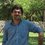Find the domain of this interesting function

What is domain of f(x)=sin(ln(x))/ln(x) ?Note by Adel Shakiba
6 years, 8 months ago

This discussion board is a place to discuss our Daily Challenges and the math and science related to those challenges. Explanations are more than just a solution — they should explain the steps and thinking strategies that you used to obtain the solution. Comments should further the discussion of math and science.

When posting on Brilliant:

• Use the emojis to react to an explanation, whether you're congratulating a job well done , or just really confused .
• Ask specific questions about the challenge or the steps in somebody's explanation. Well-posed questions can add a lot to the discussion, but posting "I don't understand!" doesn't help anyone.
• Try to contribute something new to the discussion, whether it is an extension, generalization or other idea related to the challenge.
• Stay on topic — we're all here to learn more about math and science, not to hear about your favorite get-rich-quick scheme or current world events.

MarkdownAppears as
*italics* or _italics_ italics
**bold** or __bold__ bold

- bulleted
- list

• bulleted
• list

1. numbered
2. list

1. numbered
2. list
Note: you must add a full line of space before and after lists for them to show up correctly
paragraph 1

paragraph 2

paragraph 1

paragraph 2

> This is a quote
This is a quote
# I indented these lines
# 4 spaces, and now they show
# up as a code block.

print "hello world"
# I indented these lines
# 4 spaces, and now they show
# up as a code block.

print "hello world"
MathAppears as
Remember to wrap math in $$...$$ or $...$ to ensure proper formatting.
2 \times 3 $2 \times 3$
2^{34} $2^{34}$
a_{i-1} $a_{i-1}$
\frac{2}{3} $\frac{2}{3}$
\sqrt{2} $\sqrt{2}$
\sum_{i=1}^3 $\sum_{i=1}^3$
\sin \theta $\sin \theta$
\boxed{123} $\boxed{123}$

Sort by:

I'm not sure, but I'd say $x \in R^+ - \{1\}$

- 6 years, 8 months ago

Let's wait for next answers. ;)

- 6 years, 8 months ago

Wait, by taking euler's identity into account, I think we could extend the domain further to imaginary numbers as well ..

- 6 years, 8 months ago

Please avoid taking logarithms of anything other than the positive real numbers. There is more understanding involved when we want to talk about $\log -1$, in part because it is a multi-valued function, and doesn't behave exactly like what you would expect from your experience working with $\log$ normally.

Staff - 6 years, 8 months ago

As an experience for comparing ability of mathematical software, I recommend you that try to plot this function by some popular software such as Mathlab, Maple, Derive, GeoGebra and so on. According to my experience Derive was the best for this especial function. I am waiting for the final answer.

- 6 years, 8 months ago

I plug in straight what you ask.

I'm not saying it can't be wrong, but its very rare.

- 6 years, 8 months ago

yes x>0 and x is not equal to 1

- 6 years, 8 months ago

Thank you but not complete.

- 6 years, 8 months ago

Actually, since sin (and /) are defined for (almost) all reals and ln is defined for positive reals (it might be possible to define extent ln to the imaginary numbers, but that's not exactly going to be ln any more, just like how the zeta function is not the factorial function), the domain is actually x>0, since it's defined for all x>0. Oh, excepting 1, since ln (1)=0, and division by 0 is undefined.

But we're not going to be satisfied with the domain, so let's proceed to the codomain.

Basically it has exactly the same codomain as f(x)=sin x/ x for all real values of x (since ln x has the codomain of all reals). We know that sin(x)/x-->0 as x-->0, and though it might be overkill, we can prove that sin(x)/x<1 using the Taylor series. We can then find the lower limit (as x<0) via differentiation as well*, but since that is quite tedious, and probably inexact, I have used Wolfram Alpha to estimate the lower bound at -0.217233628..., where ln (x)= 4.49340945790906...

*Set the gradient to 0 and argue that it has to lie between x= pi and x=2pi, then solve

- 6 years, 8 months ago

Thank you for your reply but I think you should look at this problem more easy.

- 6 years, 8 months ago

hello,the domain is :x belong to real such that x is greater than 1 or 0<x<1

- 6 years, 8 months ago

let's wait till next days for more answers.

- 6 years, 8 months ago